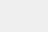# PyTorch专栏（十八）: 词嵌入，编码形式的词汇语义【磐创AI 导读】查看关于本专栏历史文章，请点击文末[阅读全文]查看本章历史文章，请点击下方蓝色字体进入相应链接阅读。

• 使用Sequence2Sequence网络和注意力进行翻译

## 词嵌入：编码形式的词汇语义代表词汇表、输入数据是维的情况下，我们往往想从神经网络中得到数据密集的结果，但是结果只有很少的几个维度（例如，预测的数据只有几个标签时）。我们如何从大的数据维度空间中得到稍小一点的维度空间？

• The mathematician ran to the store.

• The physicist ran to the store.

• The mathematician solved the open problem.

• The physicist solved the open problem.

• 我们发现数学家和物理学家在句子里有相同的作用，所以在某种程度上，他们有语义的联系。

• 当看见物理学家在新句子中的作用时，我们发现数学家也有起着相同的作用。

## 1. Getting Dense Word Embeddings（密集词嵌入）是两个向量的夹角。这就意味着，完全相似的单词相似度为1。完全不相似的单词相似度为-1。

## 2. Pytorch中的词嵌入

``# 作者: Robert Guthrie``import torch``import torch.nn as nn``import torch.nn.functional as F``import torch.optim as optim``torch.manual_seed(1)``
``word_to_ix = {"hello": 0, "world": 1}``embeds = nn.Embedding(2, 5)  # 2 words in vocab, 5 dimensional embeddings``lookup_tensor = torch.tensor([word_to_ix["hello"]], dtype=torch.long)``hello_embed = embeds(lookup_tensor)``print(hello_embed)``
• 输出结果：

``tensor([[ 0.6614,  0.2669,  0.0617,  0.6213, -0.4519]],``       grad_fn=<EmbeddingBackward>)``

## 3.例子：N-Gram语言模型是单词序列的第 i 个单词。在本例中，我们将在训练样例上计算损失函数，并且用反向传播算法更新参数。

``CONTEXT_SIZE = 2``EMBEDDING_DIM = 10``# 我们用莎士比亚的十四行诗 Sonnet 2``test_sentence = """When forty winters shall besiege thy brow,``And dig deep trenches in thy beauty's field,``Thy youth's proud livery so gazed on now,``Will be a totter'd weed of small worth held:``Then being asked, where all thy beauty lies,``Where all the treasure of thy lusty days;``To say, within thine own deep sunken eyes,``Were an all-eating shame, and thriftless praise.``How much more praise deserv'd thy beauty's use,``If thou couldst answer 'This fair child of mine``Shall sum my count, and make my old excuse,'``Proving his beauty by succession thine!``This were to be new made when thou art old,``And see thy blood warm when thou feel'st it cold.""".split()``# 应该对输入变量进行标记，但暂时忽略。``# 创建一系列的元组，每个元组都是([ word_i-2, word_i-1 ], target word)的形式。``trigrams = [([test_sentence[i], test_sentence[i + 1]], test_sentence[i + 2])``            for i in range(len(test_sentence) - 2)]``# 输出前3行，先看下是什么样子。``print(trigrams[:3])``vocab = set(test_sentence)``word_to_ix = {word: i for i, word in enumerate(vocab)}``class NGramLanguageModeler(nn.Module):``    def __init__(self, vocab_size, embedding_dim, context_size):``        super(NGramLanguageModeler, self).__init__()``        self.embeddings = nn.Embedding(vocab_size, embedding_dim)``        self.linear1 = nn.Linear(context_size * embedding_dim, 128)``        self.linear2 = nn.Linear(128, vocab_size)``    def forward(self, inputs):``        embeds = self.embeddings(inputs).view((1, -1))``        out = F.relu(self.linear1(embeds))``        out = self.linear2(out)``        log_probs = F.log_softmax(out, dim=1)``        return log_probs``losses = []``loss_function = nn.NLLLoss()``model = NGramLanguageModeler(len(vocab), EMBEDDING_DIM, CONTEXT_SIZE)``optimizer = optim.SGD(model.parameters(), lr=0.001)``for epoch in range(10):``    total_loss = 0``    for context, target in trigrams:``        # 步骤 1. 准备好进入模型的数据 (例如将单词转换成整数索引,并将其封装在变量中)``        context_idxs = torch.tensor([word_to_ix[w] for w in context], dtype=torch.long)``        # 步骤 2. 回调torch累乘梯度``        # 在传入一个新实例之前，需要把旧实例的梯度置零。``        model.zero_grad()``        # 步骤 3. 继续运行代码，得到单词的log概率值。``        log_probs = model(context_idxs)``        # 步骤 4. 计算损失函数（再次注意，Torch需要将目标单词封装在变量里）。``        loss = loss_function(log_probs, torch.tensor([word_to_ix[target]], dtype=torch.long))``        # 步骤 5. 反向传播更新梯度``        loss.backward()``        optimizer.step()``        # 通过调tensor.item()得到单个Python数值。``        total_loss += loss.item()``    losses.append(total_loss)``print(losses)  # 用训练数据每次迭代，损失函数都会下降。``
• 输出结果：

``[(['When', 'forty'], 'winters'), (['forty', 'winters'], 'shall'), (['winters', 'shall'], 'besiege')]``[523.1487259864807, 520.6150465011597, 518.0996162891388, 515.6003141403198, 513.1156675815582, 510.645352602005, 508.1888840198517, 505.74565410614014, 503.314866065979, 500.8949146270752]``

## 4.练习：计算连续词袋模型的词向量

CBOW 模型如下所示：给定一个单词代表两边的滑窗距，如，并将所有的上下文词统称为，CBOW 试图最小化• 考虑下你需要定义哪些参数。

• 确保你知道每步操作后的结构，如果想重构，请使用`.view()`

``CONTEXT_SIZE = 2  # 左右各两个词``raw_text = """We are about to study the idea of a computational process.``Computational processes are abstract beings that inhabit computers.``As they evolve, processes manipulate other abstract things called data.``The evolution of a process is directed by a pattern of rules``called a program. People create programs to direct processes. In effect,``we conjure the spirits of the computer with our spells.""".split()``# 通过对`raw_text`使用set()函数，我们进行去重操作``vocab = set(raw_text)``vocab_size = len(vocab)``word_to_ix = {word: i for i, word in enumerate(vocab)}``data = []``for i in range(2, len(raw_text) - 2):``    context = [raw_text[i - 2], raw_text[i - 1],``               raw_text[i + 1], raw_text[i + 2]]``    target = raw_text[i]``    data.append((context, target))``print(data[:5])``class CBOW(nn.Module):``    def __init__(self):``        pass``    def forward(self, inputs):``        pass``# 创建模型并且训练。这里有些函数帮你在使用模块之前制作数据。``def make_context_vector(context, word_to_ix):``    idxs = [word_to_ix[w] for w in context]``    return torch.tensor(idxs, dtype=torch.long)``make_context_vector(data, word_to_ix)  # example``

`[(['We', 'are', 'to', 'study'], 'about'), (['are', 'about', 'study', 'the'], 'to'), (['about', 'to', 'the', 'idea'], 'study'), (['to', 'study', 'idea', 'of'], 'the'), (['study', 'the', 'of', 'a'], 'idea')]`32K16薪！想转行到腾讯，多亏了这几个公众号！长按扫码，关注我们